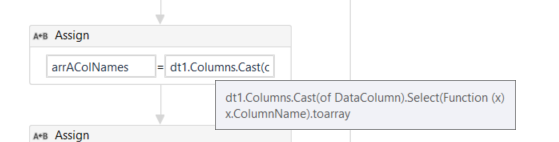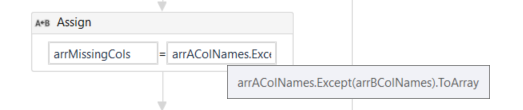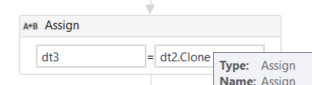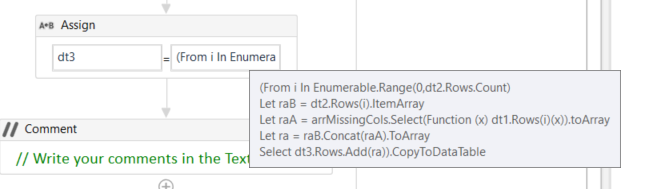# How to copy one column from DT A to another column DT B - LINQ query

Hi !

I wanted to add all datacolumns from DT A to another DT B (knowing that the columns do not exist in DT B)
I got the famous error “column name does already belong to another datatable”.
As it seems like there is no equivalent to .itemArray (that copies one row to another datatable), I could use foreach row and assign the values rows by rows, but as I might have a looooot of rows I prefer looking for a way with LINQ.

→ What I want: foreach row in a datatable, copy the data in the same index in the same column name but in another datatable.

→ What I have:

I loop in each datacolumn of A, then:

• I create an empty datacolumn in B that has the column name of A
• and I would like to call for this query. But syntaxically I am stuck:
`B_dt.AsEnumerable.Select(function(row) row(columnname).ToString = A_dt.Rows(A_dt.Rows.IndexOf(row)).Item(columnName).ToString)`

@ppr do you have an idea ?

I have:
A.xlsx (9.2 KB)
B.xlsx (8.5 KB)
and I want:
C.xlsx (8.6 KB)

Code:

1 Like

@Hiba_B

Assumptions:

• take A Col Values when Col is not present in B
• A and B DataTables have same row count

So we can implement a dynamic conditional side by side merge

Calculate the cols not present in B:prepare dt 3 and add missing cols /transfer also other col settings e.g. datatypeMerge rows:``````(From i In Enumerable.Range(0,dt2.Rows.Count)
Let raB = dt2.Rows(i).ItemArray
Let raA = arrMissingCols.Select(Function (x) dt1.Rows(i)(x)).toArray
Let ra = raB.Concat(raA).ToArray
It works fine, thank you !!!